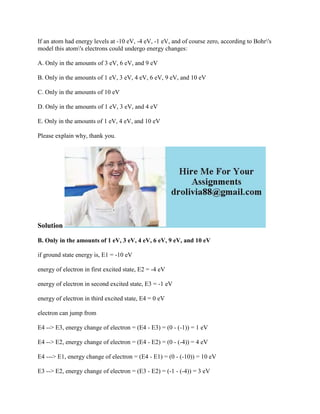Se está descargando tu SlideShare. ×

# If an atom had energy levels at -10 eV- -4 eV- -1 eV- and of course ze.docx

Anuncio
Anuncio
Anuncio
Anuncio
Anuncio
Anuncio
Anuncio
Anuncio
Anuncio
Anuncio
AnuncioCargando en…3
×

1 de 2 Anuncio

# If an atom had energy levels at -10 eV- -4 eV- -1 eV- and of course ze.docx

If an atom had energy levels at -10 eV, -4 eV, -1 eV, and of course zero, according to Bohr\'s model this atom\'s electrons could undergo energy changes:
A. Only in the amounts of 3 eV, 6 eV, and 9 eV
B. Only in the amounts of 1 eV, 3 eV, 4 eV, 6 eV, 9 eV, and 10 eV
C. Only in the amounts of 10 eV
D. Only in the amounts of 1 eV, 3 eV, and 4 eV
E. Only in the amounts of 1 eV, 4 eV, and 10 eV
Solution
B. Only in the amounts of 1 eV, 3 eV, 4 eV, 6 eV, 9 eV, and 10 eV
if ground state energy is, E1 = -10 eV
energy of electron in first excited state, E2 = -4 eV
energy of electron in second excited state, E3 = -1 eV
energy of electron in third excited state, E4 = 0 eV
electron can jump from
E4 --> E3, energy change of electron = (E4 - E3) = (0 - (-1)) = 1 eV
E4 --> E2, energy change of electron = (E4 - E2) = (0 - (-4)) = 4 eV
E4 ---> E1, energy change of electron = (E4 - E1) = (0 - (-10)) = 10 eV
E3 --> E2, energy change of electron = (E3 - E2) = (-1 - (-4)) = 3 eV
E3 --> E1, energy change of electron = (E3 - E1) = (-1 - (-10)) = 9 eV
E2 --> E1, energy change of electron = (E2 - E1) = (-4 - (-10)) = 6 eV
.

If an atom had energy levels at -10 eV, -4 eV, -1 eV, and of course zero, according to Bohr\'s model this atom\'s electrons could undergo energy changes:
A. Only in the amounts of 3 eV, 6 eV, and 9 eV
B. Only in the amounts of 1 eV, 3 eV, 4 eV, 6 eV, 9 eV, and 10 eV
C. Only in the amounts of 10 eV
D. Only in the amounts of 1 eV, 3 eV, and 4 eV
E. Only in the amounts of 1 eV, 4 eV, and 10 eV
Solution
B. Only in the amounts of 1 eV, 3 eV, 4 eV, 6 eV, 9 eV, and 10 eV
if ground state energy is, E1 = -10 eV
energy of electron in first excited state, E2 = -4 eV
energy of electron in second excited state, E3 = -1 eV
energy of electron in third excited state, E4 = 0 eV
electron can jump from
E4 --> E3, energy change of electron = (E4 - E3) = (0 - (-1)) = 1 eV
E4 --> E2, energy change of electron = (E4 - E2) = (0 - (-4)) = 4 eV
E4 ---> E1, energy change of electron = (E4 - E1) = (0 - (-10)) = 10 eV
E3 --> E2, energy change of electron = (E3 - E2) = (-1 - (-4)) = 3 eV
E3 --> E1, energy change of electron = (E3 - E1) = (-1 - (-10)) = 9 eV
E2 --> E1, energy change of electron = (E2 - E1) = (-4 - (-10)) = 6 eV
.

Anuncio
Anuncio

### If an atom had energy levels at -10 eV- -4 eV- -1 eV- and of course ze.docx

1. 1. If an atom had energy levels at -10 eV, -4 eV, -1 eV, and of course zero, according to Bohr's model this atom's electrons could undergo energy changes: A. Only in the amounts of 3 eV, 6 eV, and 9 eV B. Only in the amounts of 1 eV, 3 eV, 4 eV, 6 eV, 9 eV, and 10 eV C. Only in the amounts of 10 eV D. Only in the amounts of 1 eV, 3 eV, and 4 eV E. Only in the amounts of 1 eV, 4 eV, and 10 eV Please explain why, thank you. Solution B. Only in the amounts of 1 eV, 3 eV, 4 eV, 6 eV, 9 eV, and 10 eV if ground state energy is, E1 = -10 eV energy of electron in first excited state, E2 = -4 eV energy of electron in second excited state, E3 = -1 eV energy of electron in third excited state, E4 = 0 eV electron can jump from E4 --> E3, energy change of electron = (E4 - E3) = (0 - (-1)) = 1 eV E4 --> E2, energy change of electron = (E4 - E2) = (0 - (-4)) = 4 eV E4 ---> E1, energy change of electron = (E4 - E1) = (0 - (-10)) = 10 eV E3 --> E2, energy change of electron = (E3 - E2) = (-1 - (-4)) = 3 eV
2. 2. E3 --> E1, energy change of electron = (E3 - E1) = (-1 - (-10)) = 9 eV E2 --> E1, energy change of electron = (E2 - E1) = (-4 - (-10)) = 6 eV